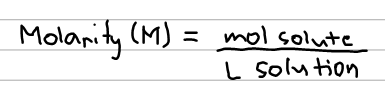# Problem: An aqueous KNO3 solution is made using 77.6 g of KNO3 diluted to a total solution volume of 1.96 L. (Assume a density of 1.05 g/mL for the solution)Calculate the molarity of the solution.

###### FREE Expert Solution

The molarity of the solution can be calculated using the equation below:Since we are only given the mass of the solute, we still have to calculate for moles and will have to go through multiple steps.

86% (399 ratings)###### Problem Details
An aqueous KNO3 solution is made using 77.6 g of KNO3 diluted to a total solution volume of 1.96 L. (Assume a density of 1.05 g/mL for the solution)

Calculate the molarity of the solution.